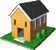Ontario Research Centre for Computer Algebra Technical Reports
The Ontario Research Centre for Computer Algebra

# The UWO ORCCA Reading Room

## UWO ORCCA TR-03-04 Summary

Determination of approximate symmetries of differential equations, November, 2003, Joe Bonasia, Francois Lemaire, Greg Reid and Lihong Zhi, 16 pages

Abstract: There has been considerable progress in the theory and computer implementation of symbolic computation algorithms to automatically determine and exploit exact symmetries of exact differential equations. Such programs usually apply a finite number of exact differentiations and eliminations to the overdetermined linearized equations for the unknown symmetries (the symmetry defining equations), to complete them to certain involutive or standard forms. The symmetry properties can be determined from these involutive forms.

In many applications, however, the differential equations describing a model are only known approximately. For example they may contain parameters that are only known approximately. Symbolic methods are unstable if applied to the symmetry defining equations directly, and indirect techniques (e.g. replacing approximate parameters by symbolic ones) might not be practical in cases with many parameters. Discussion and examples are given of such difficulties.

A new generation of symbolic-numeric methods is described and applied to the problem of determining symmetries of differential equations.

We introduce a class of differential-elimination methods which uses Numerical Linear Algebra, and in particular the Singular Value Decomposition, to perform the elimination process on the symmetry defining equations. Our approach uses symbolic differentiations but not symbolic eliminations. Substitution of an appropriate random point in the independent variables, followed by numerical projection is used to test for the conditions of completion to a projective involutive form. We prove that this form is equivalent to the involutive form of the Cartan-Kuranishi theory of partial differential equations.

Our method is applied to determining symmetry properties of 50 {\sc ode} from the collection in Kamke's book.

 About ORCCA Members Events & SeminarsORCCA Home Research Activities Positions Reading Room Contact Info